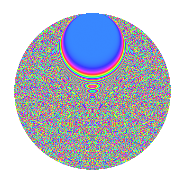# Properties

 Label 1849.2.iLevel 1849 Weight 2 Character orbit i Rep. character $$\chi_{1849}(44,\cdot)$$ Character field $$\Q(\zeta_{43})$$ Dimension 6552 Sturm bound 315

# Related objects

## Defining parameters

 Level: $$N$$ $$=$$ $$1849 = 43^{2}$$ Weight: $$k$$ $$=$$ $$2$$ Character orbit: $$[\chi]$$ $$=$$ 1849.i (of order $$43$$ and degree $$42$$) Character conductor: $$\operatorname{cond}(\chi)$$ $$=$$ $$1849$$ Character field: $$\Q(\zeta_{43})$$ Sturm bound: $$315$$

## Dimensions

The following table gives the dimensions of various subspaces of $$M_{2}(1849, [\chi])$$.

Total New Old
Modular forms 6636 6636 0
Cusp forms 6552 6552 0
Eisenstein series 84 84 0

## Trace form

 $$6552q - 38q^{2} - 37q^{3} - 192q^{4} - 37q^{5} - 31q^{6} - 31q^{7} - 28q^{8} - 183q^{9} + O(q^{10})$$ $$6552q - 38q^{2} - 37q^{3} - 192q^{4} - 37q^{5} - 31q^{6} - 31q^{7} - 28q^{8} - 183q^{9} - 29q^{10} - 33q^{11} - 11q^{12} - 27q^{13} - 66q^{14} - 31q^{15} - 154q^{16} - 33q^{17} - 2q^{18} - 232q^{19} + 7q^{20} - 7q^{21} - 9q^{22} - 107q^{23} + 9q^{24} - 179q^{25} - 19q^{26} - 7q^{27} + 13q^{28} - 7q^{29} - 248q^{30} - 91q^{31} + 12q^{32} + 19q^{33} - 3q^{34} - 155q^{35} - 108q^{36} - 5q^{37} - 65q^{38} + 11q^{39} + 39q^{40} - 5q^{41} + 45q^{42} - q^{43} + 31q^{44} + 43q^{45} + 43q^{46} - 15q^{47} + 73q^{48} - 131q^{49} + 88q^{50} + 31q^{51} + 61q^{52} - 93q^{53} + 55q^{54} + 53q^{55} + 71q^{56} - 104q^{57} + 23q^{58} + 29q^{59} + 95q^{60} + 9q^{61} + 51q^{62} + 57q^{63} - 78q^{64} + 25q^{65} - 1133q^{66} + 25q^{67} - 474q^{68} + 35q^{69} + 85q^{70} + 39q^{71} - 450q^{72} + 53q^{73} + 81q^{74} - 558q^{75} - 71q^{76} + 41q^{77} + 106q^{78} + 37q^{79} + 143q^{80} - 55q^{81} - 415q^{82} + 7q^{83} + 167q^{84} + 41q^{85} + 84q^{86} - 535q^{87} + 153q^{88} + 63q^{89} - 605q^{90} + 65q^{91} + 123q^{92} + 83q^{93} - 407q^{94} - 156q^{95} + 211q^{96} - 514q^{97} + 130q^{98} - 112q^{99} + O(q^{100})$$

## Decomposition of $$S_{2}^{\mathrm{new}}(1849, [\chi])$$ into newform subspaces

The newforms in this space have not yet been added to the LMFDB.

## Hecke characteristic polynomials

There are no characteristic polynomials of Hecke operators in the database